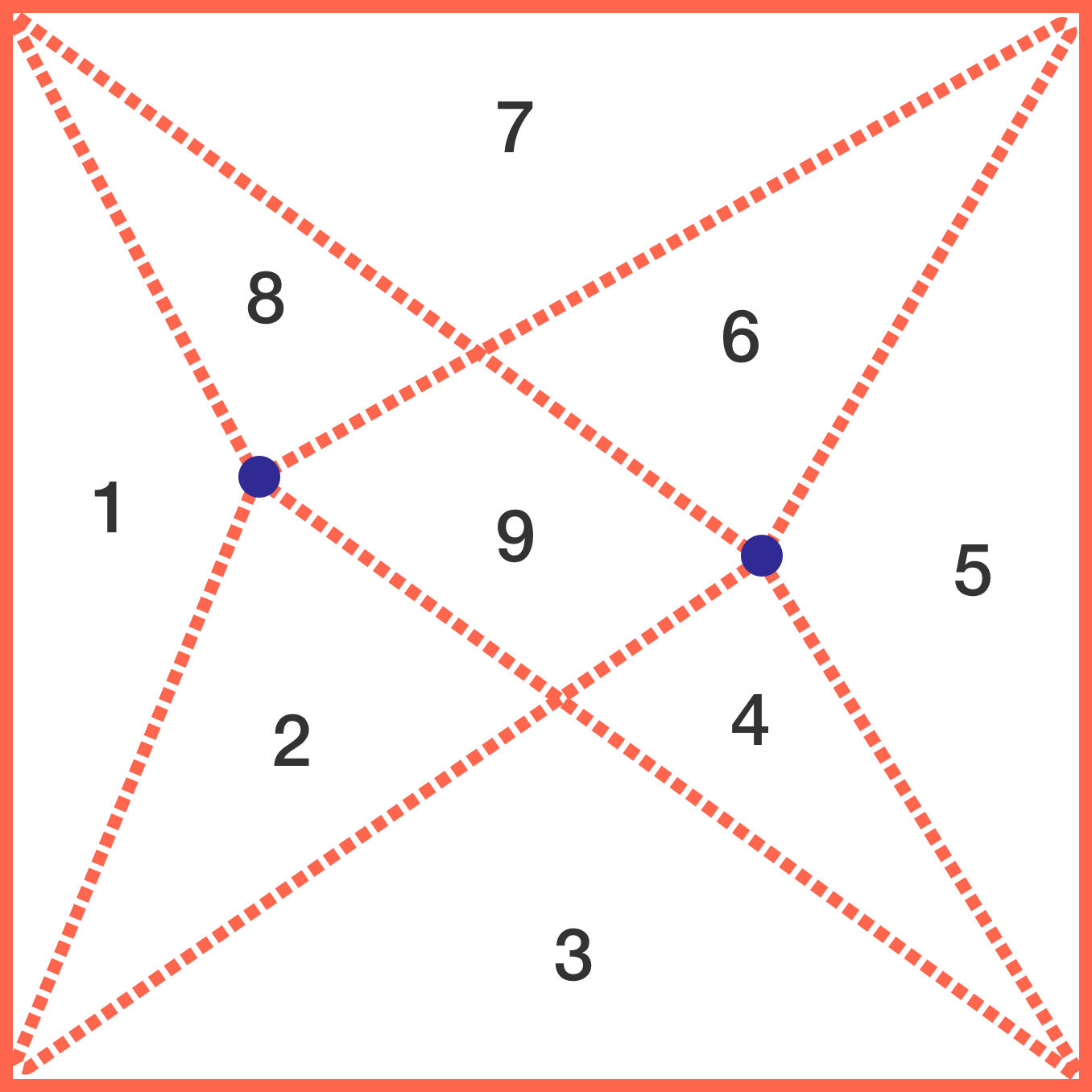# Nine!

Geometry Level 2

Choose two points--for example, the 2 blue points in the diagram--inside a square with area 9, such that drawing a line segment between each of these 2 points and each vertex of the square divides the square into 9 parts.

Is it possible that all of the 9 parts have an equal area of 1?×